# Mean State

Period Mean (original grids) [W/m2]
Model Period Mean (intersection) [W/m2]
Model Period Mean (complement) [W/m2]
Benchmark Period Mean (intersection) [W/m2]
Benchmark Period Mean (complement) [W/m2]
Bias [W/m2]
RMSE [W/m2]
Phase Shift [months]
Bias Score 
RMSE Score 
Seasonal Cycle Score 
Spatial Distribution Score 
Interannual Variability Score 
Overall Score 
Benchmark [-] 187.
CRUNCEPv7 [-] 192. 192. 0.00 186. 206. 5.63 16.6 1.10 0.62 0.49 0.86 0.95 0.48 0.65
GSWP3v1 [-] 182. 182. 0.00 186. 206. -4.31 15.3 0.775 0.73 0.49 0.91 0.92 0.80 0.72
WFDEI [-] 167. 167. 0.00 186. 206. -18.6 28.6 1.40 0.39 0.37 0.80 0.95 0.78 0.61
Period Mean (original grids) [W/m2]
Model Period Mean (intersection) [W/m2]
Model Period Mean (complement) [W/m2]
Benchmark Period Mean (intersection) [W/m2]
Benchmark Period Mean (complement) [W/m2]
Bias [W/m2]
RMSE [W/m2]
Phase Shift [months]
Bias Score 
RMSE Score 
Seasonal Cycle Score 
Spatial Distribution Score 
Interannual Variability Score 
Overall Score 
Benchmark [-] 198.
CRUNCEPv7 [-] 198. 198. 0.00 198. 214. 0.100 18.7 1.51 0.57 0.47 0.78 0.93 0.72 0.66
GSWP3v1 [-] 194. 194. 0.00 198. 214. -3.39 14.2 0.702 0.72 0.51 0.92 0.98 0.83 0.75
WFDEI [-] 190. 190. 0.00 198. 214. -7.32 20.3 0.916 0.55 0.45 0.90 0.99 0.81 0.69
Period Mean (original grids) [W/m2]
Model Period Mean (intersection) [W/m2]
Model Period Mean (complement) [W/m2]
Benchmark Period Mean (intersection) [W/m2]
Benchmark Period Mean (complement) [W/m2]
Bias [W/m2]
RMSE [W/m2]
Phase Shift [months]
Bias Score 
RMSE Score 
Seasonal Cycle Score 
Spatial Distribution Score 
Interannual Variability Score 
Overall Score 
Benchmark [-] 199.
CRUNCEPv7 [-] 195. 195. 0.00 198. 219. -2.87 17.1 1.13 0.71 0.56 0.84 0.85 0.53 0.67
GSWP3v1 [-] 196. 197. 0.00 198. 219. -2.35 15.5 0.774 0.80 0.57 0.89 0.99 0.74 0.76
WFDEI [-] 196. 196. 0.00 198. 219. -2.31 21.1 1.13 0.71 0.47 0.83 0.99 0.73 0.70
Period Mean (original grids) [W/m2]
Model Period Mean (intersection) [W/m2]
Model Period Mean (complement) [W/m2]
Benchmark Period Mean (intersection) [W/m2]
Benchmark Period Mean (complement) [W/m2]
Bias [W/m2]
RMSE [W/m2]
Phase Shift [months]
Bias Score 
RMSE Score 
Seasonal Cycle Score 
Spatial Distribution Score 
Interannual Variability Score 
Overall Score 
Benchmark [-] 70.7
CRUNCEPv7 [-] 72.8 72.9 0.00 70.5 72.8 1.13 20.4 0.333 0.91 0.75 0.98 1.0 0.71 0.85
GSWP3v1 [-] 70.3 70.4 0.00 70.5 72.8 -0.648 18.5 0.319 0.93 0.76 0.98 1.0 0.76 0.87
WFDEI [-] 60.0 60.2 0.00 70.5 72.8 -10.7 23.4 0.382 0.85 0.73 0.97 0.99 0.85 0.85
Period Mean (original grids) [W/m2]
Model Period Mean (intersection) [W/m2]
Model Period Mean (complement) [W/m2]
Benchmark Period Mean (intersection) [W/m2]
Benchmark Period Mean (complement) [W/m2]
Bias [W/m2]
RMSE [W/m2]
Phase Shift [months]
Bias Score 
RMSE Score 
Seasonal Cycle Score 
Spatial Distribution Score 
Interannual Variability Score 
Overall Score 
Benchmark [-] 194.
CRUNCEPv7 [-] 187. 187. 0.00 190. 207. -0.526 18.0 1.52 0.61 0.44 0.77 0.84 0.52 0.60
GSWP3v1 [-] 176. 176. 0.00 190. 207. -10.3 18.7 1.27 0.57 0.44 0.80 0.96 0.77 0.66
WFDEI [-] 165. 165. 0.00 190. 207. -21.0 28.1 1.67 0.36 0.39 0.74 0.99 0.69 0.59
Period Mean (original grids) [W/m2]
Model Period Mean (intersection) [W/m2]
Model Period Mean (complement) [W/m2]
Benchmark Period Mean (intersection) [W/m2]
Benchmark Period Mean (complement) [W/m2]
Bias [W/m2]
RMSE [W/m2]
Phase Shift [months]
Bias Score 
RMSE Score 
Seasonal Cycle Score 
Spatial Distribution Score 
Interannual Variability Score 
Overall Score 
Benchmark [-] 97.8
CRUNCEPv7 [-] 109. 109. 0.00 97.6 118. 11.2 20.9 0.260 0.84 0.79 0.98 1.0 0.72 0.85
GSWP3v1 [-] 102. 102. 0.00 97.6 118. 4.51 14.6 0.227 0.93 0.83 0.98 0.99 0.67 0.87
WFDEI [-] 94.7 94.7 0.00 97.6 118. -2.51 18.3 0.358 0.93 0.77 0.98 0.98 0.80 0.87
Period Mean (original grids) [W/m2]
Model Period Mean (intersection) [W/m2]
Model Period Mean (complement) [W/m2]
Benchmark Period Mean (intersection) [W/m2]
Benchmark Period Mean (complement) [W/m2]
Bias [W/m2]
RMSE [W/m2]
Phase Shift [months]
Bias Score 
RMSE Score 
Seasonal Cycle Score 
Spatial Distribution Score 
Interannual Variability Score 
Overall Score 
Benchmark [-] 139.
CRUNCEPv7 [-] 149. 149. 0.00 138. 149. 11.1 23.8 0.446 0.75 0.74 0.96 0.97 0.72 0.81
GSWP3v1 [-] 136. 136. 0.00 138. 149. -2.20 15.9 0.348 0.88 0.77 0.97 1.0 0.67 0.84
WFDEI [-] 142. 141. 0.00 138. 149. 3.22 23.6 0.527 0.83 0.68 0.95 0.95 0.77 0.81
Period Mean (original grids) [W/m2]
Model Period Mean (intersection) [W/m2]
Model Period Mean (complement) [W/m2]
Benchmark Period Mean (intersection) [W/m2]
Benchmark Period Mean (complement) [W/m2]
Bias [W/m2]
RMSE [W/m2]
Phase Shift [months]
Bias Score 
RMSE Score 
Seasonal Cycle Score 
Spatial Distribution Score 
Interannual Variability Score 
Overall Score 
Benchmark [-] 181.
CRUNCEPv7 [-] 194. 194. 0.00 179. 205. 14.7 26.0 0.341 0.57 0.58 0.97 0.96 0.65 0.72
GSWP3v1 [-] 175. 175. 0.00 179. 205. -3.00 18.1 0.342 0.78 0.61 0.97 0.95 0.72 0.77
WFDEI [-] 175. 175. 0.00 179. 205. -3.64 24.6 0.502 0.69 0.52 0.96 1.0 0.71 0.73
Period Mean (original grids) [W/m2]
Model Period Mean (intersection) [W/m2]
Model Period Mean (complement) [W/m2]
Benchmark Period Mean (intersection) [W/m2]
Benchmark Period Mean (complement) [W/m2]
Bias [W/m2]
RMSE [W/m2]
Phase Shift [months]
Bias Score 
RMSE Score 
Seasonal Cycle Score 
Spatial Distribution Score 
Interannual Variability Score 
Overall Score 
Benchmark [-] 62.8
CRUNCEPv7 [-] 64.2 64.7 0.00 63.2 54.4 0.281 20.6 0.281 0.92 0.73 0.98 0.96 0.75 0.85
GSWP3v1 [-] 61.3 61.7 0.00 63.2 54.4 -2.47 16.4 0.176 0.94 0.78 0.99 0.98 0.76 0.87
WFDEI [-] 50.5 51.0 0.00 63.2 54.4 -13.1 24.3 0.308 0.81 0.72 0.98 0.99 0.85 0.85
Period Mean (original grids) [W/m2]
Model Period Mean (intersection) [W/m2]
Model Period Mean (complement) [W/m2]
Benchmark Period Mean (intersection) [W/m2]
Benchmark Period Mean (complement) [W/m2]
Bias [W/m2]
RMSE [W/m2]
Phase Shift [months]
Bias Score 
RMSE Score 
Seasonal Cycle Score 
Spatial Distribution Score 
Interannual Variability Score 
Overall Score 
Benchmark [-] 198.
CRUNCEPv7 [-] 194. 194. 0.00 198. 197. -5.32 15.0 0.501 0.84 0.78 0.97 0.97 0.65 0.83
GSWP3v1 [-] 190. 190. 0.00 198. 197. -9.01 16.6 0.371 0.82 0.76 0.97 1.0 0.71 0.84
WFDEI [-] 194. 194. 0.00 198. 197. -5.97 19.9 0.422 0.86 0.68 0.97 1.0 0.81 0.83
Period Mean (original grids) [W/m2]
Model Period Mean (intersection) [W/m2]
Model Period Mean (complement) [W/m2]
Benchmark Period Mean (intersection) [W/m2]
Benchmark Period Mean (complement) [W/m2]
Bias [W/m2]
RMSE [W/m2]
Phase Shift [months]
Bias Score 
RMSE Score 
Seasonal Cycle Score 
Spatial Distribution Score 
Interannual Variability Score 
Overall Score 
Benchmark [-] 120.
CRUNCEPv7 [-] 133. 133. 0.00 118. 145. 15.5 24.9 0.231 0.76 0.80 0.98 0.99 0.73 0.85
GSWP3v1 [-] 114. 114. 0.00 118. 145. -1.47 13.2 0.209 0.93 0.84 0.99 0.99 0.66 0.87
WFDEI [-] 113. 113. 0.00 118. 145. -2.40 20.1 0.364 0.91 0.76 0.98 1.0 0.83 0.87
Period Mean (original grids) [W/m2]
Model Period Mean (intersection) [W/m2]
Model Period Mean (complement) [W/m2]
Benchmark Period Mean (intersection) [W/m2]
Benchmark Period Mean (complement) [W/m2]
Bias [W/m2]
RMSE [W/m2]
Phase Shift [months]
Bias Score 
RMSE Score 
Seasonal Cycle Score 
Spatial Distribution Score 
Interannual Variability Score 
Overall Score 
Benchmark [-] 163.
CRUNCEPv7 [-] 144. 144. 0.00 142. 173. 1.83 19.8 0.580 0.75 0.69 0.93 0.99 0.61 0.78
GSWP3v1 [-] 137. 137. 0.00 142. 173. -4.46 16.0 0.389 0.82 0.70 0.96 1.0 0.71 0.82
WFDEI [-] 136. 136. 0.00 142. 173. -5.53 21.5 0.584 0.75 0.64 0.93 1.0 0.75 0.78
Period Mean (original grids) [W/m2]
Model Period Mean (intersection) [W/m2]
Model Period Mean (complement) [W/m2]
Benchmark Period Mean (intersection) [W/m2]
Benchmark Period Mean (complement) [W/m2]
Bias [W/m2]
RMSE [W/m2]
Phase Shift [months]
Bias Score 
RMSE Score 
Seasonal Cycle Score 
Spatial Distribution Score 
Interannual Variability Score 
Overall Score 
Benchmark [-] 177.
CRUNCEPv7 [-] 168. 168. 0.00 175. 217. -6.22 17.9 0.405 0.72 0.75 0.96 0.98 0.71 0.81
GSWP3v1 [-] 162. 162. 0.00 175. 217. -12.0 17.9 0.298 0.71 0.76 0.98 0.96 0.67 0.81
WFDEI [-] 172. 172. 0.00 175. 217. -2.53 16.8 0.507 0.78 0.73 0.96 0.98 0.65 0.80
Period Mean (original grids) [W/m2]
Model Period Mean (intersection) [W/m2]
Model Period Mean (complement) [W/m2]
Benchmark Period Mean (intersection) [W/m2]
Benchmark Period Mean (complement) [W/m2]
Bias [W/m2]
RMSE [W/m2]
Phase Shift [months]
Bias Score 
RMSE Score 
Seasonal Cycle Score 
Spatial Distribution Score 
Interannual Variability Score 
Overall Score 
Benchmark [-] 69.7
CRUNCEPv7 [-] 74.1 74.0 0.00 69.6 71.1 2.14 20.6 0.113 0.89 0.75 0.99 0.93 0.62 0.82
GSWP3v1 [-] 69.2 69.2 0.00 69.6 71.1 -1.83 17.2 0.0813 0.92 0.78 0.99 0.99 0.76 0.87
WFDEI [-] 62.0 61.9 0.00 69.6 71.1 -9.33 23.4 0.141 0.85 0.73 0.99 0.95 0.80 0.84
Period Mean (original grids) [W/m2]
Model Period Mean (intersection) [W/m2]
Model Period Mean (complement) [W/m2]
Benchmark Period Mean (intersection) [W/m2]
Benchmark Period Mean (complement) [W/m2]
Bias [W/m2]
RMSE [W/m2]
Phase Shift [months]
Bias Score 
RMSE Score 
Seasonal Cycle Score 
Spatial Distribution Score 
Interannual Variability Score 
Overall Score 
Benchmark [-] 204.
CRUNCEPv7 [-] 190. 190. 0.00 201. 219. -8.69 24.0 0.969 0.61 0.64 0.90 0.82 0.67 0.71
GSWP3v1 [-] 192. 192. 0.00 201. 219. -6.68 16.7 0.458 0.77 0.68 0.94 0.99 0.75 0.80
WFDEI [-] 194. 194. 0.00 201. 219. -6.90 21.4 0.489 0.72 0.61 0.94 1.0 0.70 0.76
Period Mean (original grids) [W/m2]
Model Period Mean (intersection) [W/m2]
Model Period Mean (complement) [W/m2]
Benchmark Period Mean (intersection) [W/m2]
Benchmark Period Mean (complement) [W/m2]
Bias [W/m2]
RMSE [W/m2]
Phase Shift [months]
Bias Score 
RMSE Score 
Seasonal Cycle Score 
Spatial Distribution Score 
Interannual Variability Score 
Overall Score 
Benchmark [-] 150.
CRUNCEPv7 [-] 155. 156. 0.00 148. 192. 8.22 18.2 0.417 0.83 0.78 0.97 0.99 0.58 0.82
GSWP3v1 [-] 145. 145. 0.00 148. 192. -1.45 13.3 0.293 0.94 0.80 0.98 0.99 0.70 0.87
WFDEI [-] 150. 150. 0.00 148. 192. 3.00 22.3 0.394 0.90 0.69 0.97 1.0 0.84 0.85
Period Mean (original grids) [W/m2]
Model Period Mean (intersection) [W/m2]
Model Period Mean (complement) [W/m2]
Benchmark Period Mean (intersection) [W/m2]
Benchmark Period Mean (complement) [W/m2]
Bias [W/m2]
RMSE [W/m2]
Phase Shift [months]
Bias Score 
RMSE Score 
Seasonal Cycle Score 
Spatial Distribution Score 
Interannual Variability Score 
Overall Score 
Benchmark [-] 110.
CRUNCEPv7 [-] 122. 123. 0.00 110. 117. 12.7 21.9 0.218 0.83 0.80 0.99 0.97 0.56 0.82
GSWP3v1 [-] 112. 112. 0.00 110. 117. 1.79 13.4 0.112 0.93 0.84 0.99 1.0 0.68 0.88
WFDEI [-] 111. 111. 0.00 110. 117. 1.60 19.6 0.186 0.91 0.77 0.99 0.94 0.84 0.87
Period Mean (original grids) [W/m2]
Model Period Mean (intersection) [W/m2]
Model Period Mean (complement) [W/m2]
Benchmark Period Mean (intersection) [W/m2]
Benchmark Period Mean (complement) [W/m2]
Bias [W/m2]
RMSE [W/m2]
Phase Shift [months]
Bias Score 
RMSE Score 
Seasonal Cycle Score 
Spatial Distribution Score 
Interannual Variability Score 
Overall Score 
Benchmark [-] 161.
CRUNCEPv7 [-] 155. 155. 0.00 161. 163. -5.79 16.7 0.204 0.88 0.81 0.99 0.94 0.61 0.84
GSWP3v1 [-] 160. 160. 0.00 161. 163. -0.462 14.3 0.198 0.93 0.83 0.99 0.98 0.70 0.88
WFDEI [-] 169. 169. 0.00 161. 163. 8.11 23.2 0.247 0.88 0.74 0.98 1.0 0.84 0.86
Period Mean (original grids) [W/m2]
Model Period Mean (intersection) [W/m2]
Model Period Mean (complement) [W/m2]
Benchmark Period Mean (intersection) [W/m2]
Benchmark Period Mean (complement) [W/m2]
Bias [W/m2]
RMSE [W/m2]
Phase Shift [months]
Bias Score 
RMSE Score 
Seasonal Cycle Score 
Spatial Distribution Score 
Interannual Variability Score 
Overall Score 
Benchmark [-] 179.
CRUNCEPv7 [-] 183. 183. 0.00 178. 218. 6.21 17.3 1.49 0.60 0.45 0.78 0.82 0.58 0.61
GSWP3v1 [-] 174. 174. 0.00 178. 218. -3.01 14.3 0.799 0.75 0.45 0.90 0.96 0.81 0.72
WFDEI [-] 160. 160. 0.00 178. 218. -17.7 27.4 1.47 0.35 0.35 0.80 0.99 0.64 0.58
Period Mean (original grids) [W/m2]
Model Period Mean (intersection) [W/m2]
Model Period Mean (complement) [W/m2]
Benchmark Period Mean (intersection) [W/m2]
Benchmark Period Mean (complement) [W/m2]
Bias [W/m2]
RMSE [W/m2]
Phase Shift [months]
Bias Score 
RMSE Score 
Seasonal Cycle Score 
Spatial Distribution Score 
Interannual Variability Score 
Overall Score 
Benchmark [-] 185.
CRUNCEPv7 [-] 187. 187. 0.00 184. 202. 2.66 20.0 0.995 0.70 0.62 0.88 0.82 0.52 0.69
GSWP3v1 [-] 180. 181. 0.00 184. 202. -3.37 15.5 0.717 0.83 0.64 0.91 0.98 0.72 0.79
WFDEI [-] 175. 175. 0.00 184. 202. -8.85 23.8 1.03 0.71 0.53 0.87 0.96 0.78 0.73
Period Mean (original grids) [W/m2]
Model Period Mean (intersection) [W/m2]
Model Period Mean (complement) [W/m2]
Benchmark Period Mean (intersection) [W/m2]
Benchmark Period Mean (complement) [W/m2]
Bias [W/m2]
RMSE [W/m2]
Phase Shift [months]
Bias Score 
RMSE Score 
Seasonal Cycle Score 
Spatial Distribution Score 
Interannual Variability Score 
Overall Score 
Benchmark [-] 178.
CRUNCEPv7 [-] 179. 178. 0.00 179. 169. -3.51 27.2 0.548 0.69 0.69 0.95 0.80 0.63 0.74
GSWP3v1 [-] 177. 176. 0.00 179. 169. -7.13 20.8 0.400 0.79 0.71 0.96 1.0 0.69 0.81
WFDEI [-] 182. 181. 0.00 179. 169. -2.00 24.2 0.510 0.77 0.68 0.95 0.99 0.72 0.80

# Temporally integrated period mean

BENCHMARK MEAN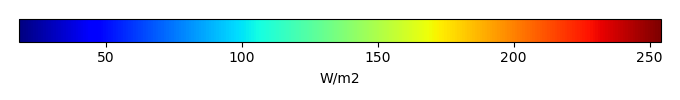MODEL MEANBIAS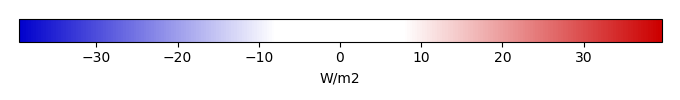BIAS SCORERMSE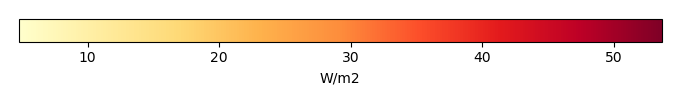RMSE SCOREBENCHMARK INTERANNUAL VARIABILITY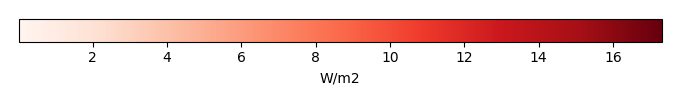MODEL INTERANNUAL VARIABILITYINTERANNUAL VARIABILITY SCOREBENCHMARK MAX MONTHMODEL MAX MONTHDIFFERENCE IN MAX MONTH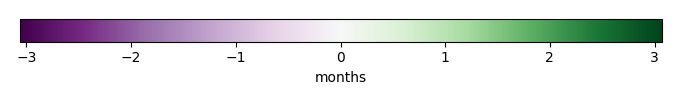SEASONAL CYCLE SCORESPATIAL TAYLOR DIAGRAMMODEL COLORS# Spatially integrated regional mean

MODEL COLORSREGIONAL MEANANNUAL CYCLEMONTHLY ANOMALYANNUAL CYCLE# All Models

BenchmarkCRUNCEPv7GSWP3v1WFDEI# Data Information

creation_date: Thu May 8 23:03:44 PDT 2014

source_file: This product is generated from monthly 1 degree CERES EBAF Radiation observations